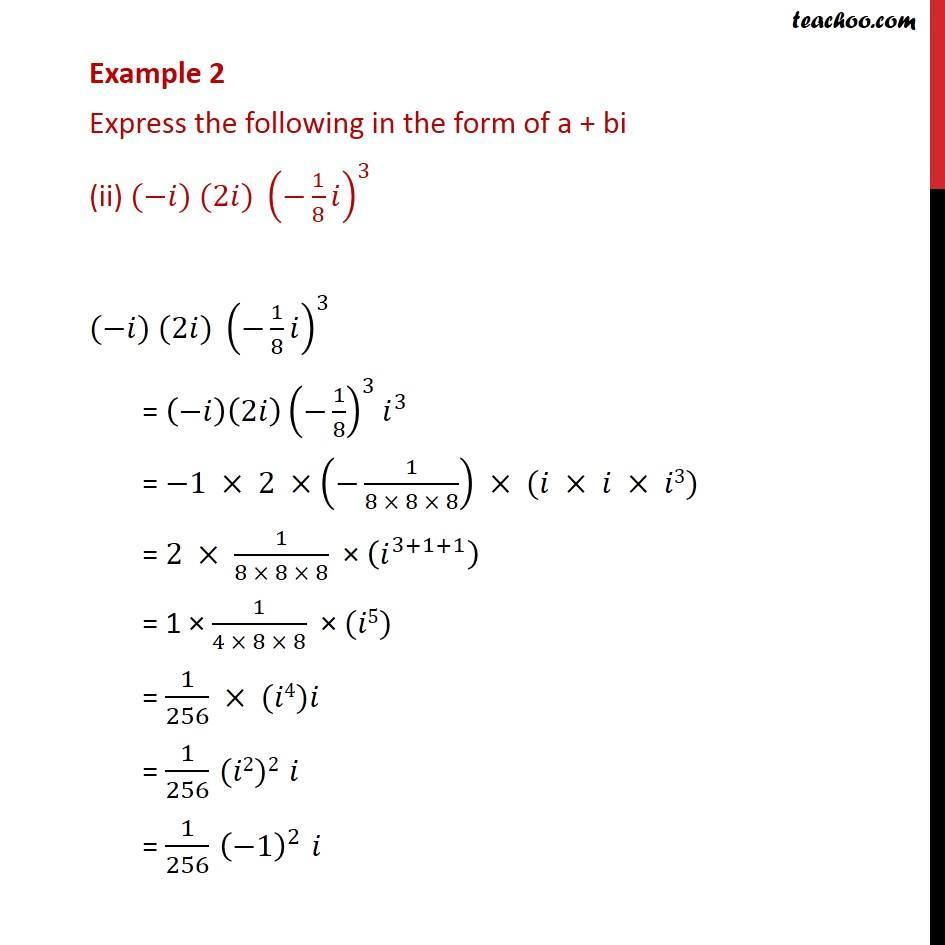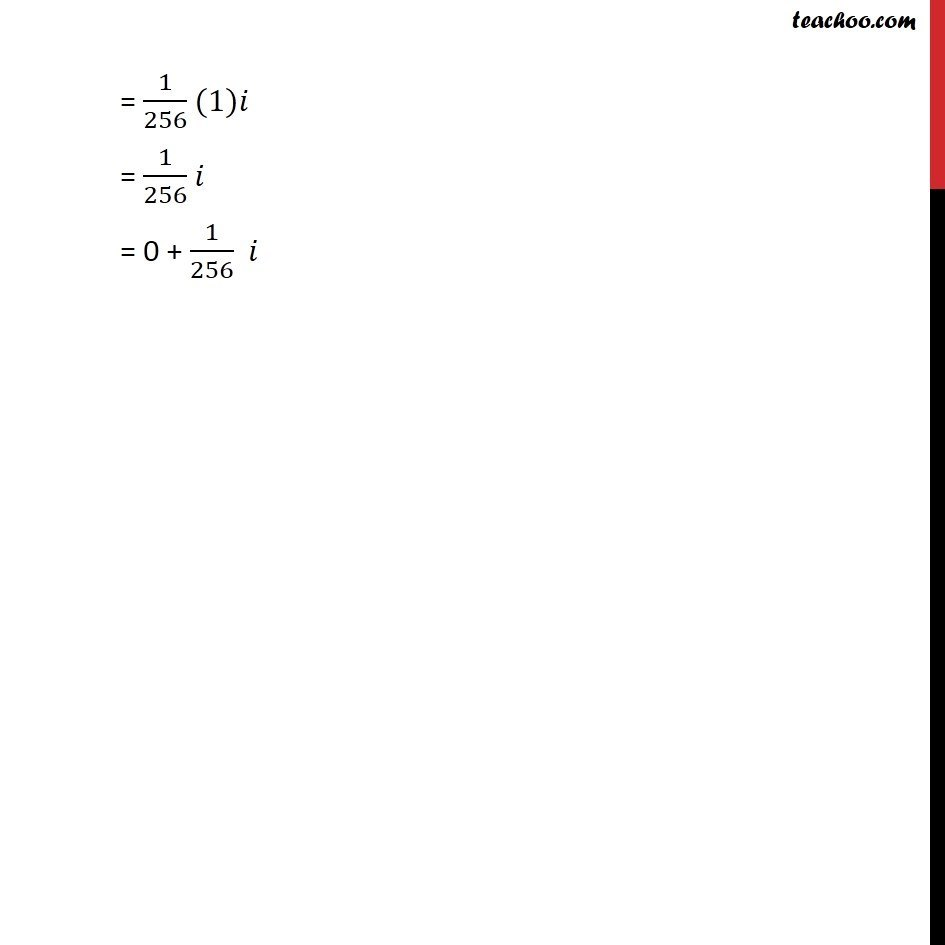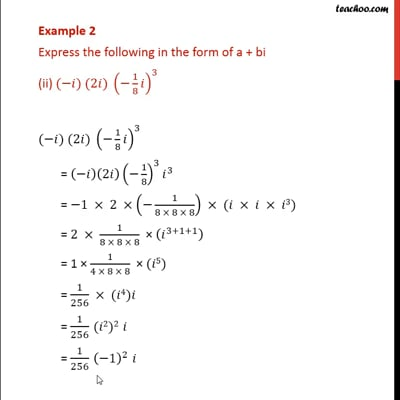Examples

Chapter 5 Class 11 Complex Numbers
Serial order wiseThis video is only available for Teachoo black users

Maths Crash Course - Live lectures + all videos + Real time Doubt solving!

### Transcript

Example 2 Express the following in the form of a + bi (ii) ( ) (2 ) ( 1/8 )^3 ( ) (2 ) ( 1/8 )^3 = ( )(2 ) ( 1/8)^3 ^3 = 1 2 ( 1/(8 8 8)) ( 3) = 2 1/(8 8 8) ( ^(3+1+1) ) = 1 1/(4 8 8) ( 5) = 1/256 ( 4) = 1/256 ( 2)2 = 1/256 ( 1)^2 = 1/256 (1) = 1/256 = 0 + 1/256